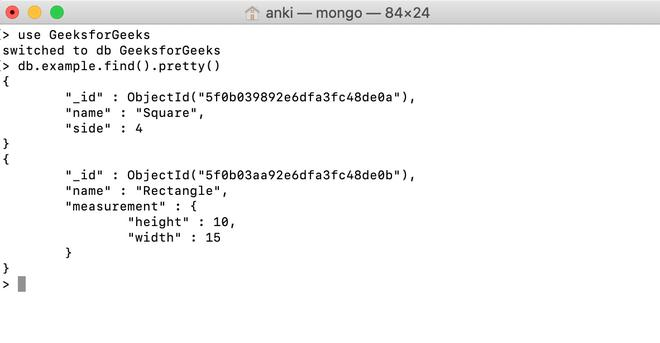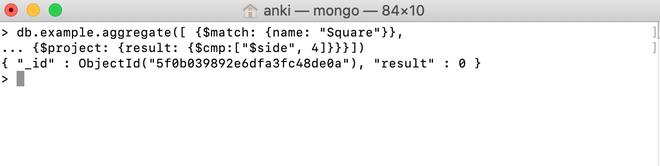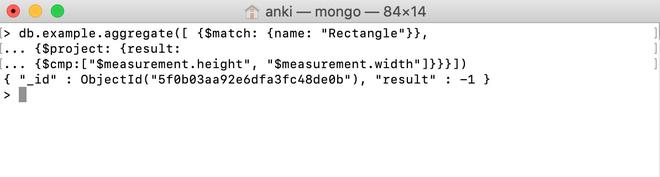# MongoDB \$cmp Operator

MongoDB provides different types of comparison expression operators that are used in the aggregation pipeline stages \$cmp operator is one of them. This operator is used to perform a comparison between two values and return the following result according to the condition:

• If the first value is greater than the second value, then this operator will return 1
• If the first value is less than the second value, then this operator will return -1
• If both the values are equal, then this operator will return 0.

Syntax:

```{ \$cmp: [ <expression1>, <expression2> ] }

```

Examples:

In the following examples, we are working with:

Database: GeeksforGeeks

Collection: example

Document: two documents that contain the details of the shapes in the form of field-value pairs.Using \$cmp Operator:

In this example, we are comparing the value of the side field with 4 and \$cmp operator return 0 which means both values are equal.

```db.example.aggregate([{\$match: {name: "Square"}},
... {\$project: {result: {\$cmp:["\$side", 4]}}}])```Using \$cmp Operator in the Embedded Document:

In this example, we are comparing the value of the measurement.height field with the value of the measurement.width field and \$cmp operator return -1 which means both values of measurement.height field is less than the value of the measurement.width field.

```db.example.aggregate([{\$match: {name: "Rectangle"}},
... {\$project: {result:
... {\$cmp:["\$measurement.height", "\$measurement.width"]}}}])```My Personal Notes arrow_drop_upCheck out this Author's contributed articles.

If you like GeeksforGeeks and would like to contribute, you can also write an article using contribute.geeksforgeeks.org or mail your article to contribute@geeksforgeeks.org. See your article appearing on the GeeksforGeeks main page and help other Geeks.

Please Improve this article if you find anything incorrect by clicking on the "Improve Article" button below.

Article Tags :

Be the First to upvote.

Please write to us at contribute@geeksforgeeks.org to report any issue with the above content.